#### 期刊菜单

The Study on Smooth Spline Regressionand Its Application
DOI: 10.12677/SA.2019.84069, PDF, HTML, XML, 下载: 1,329  浏览: 4,432

Abstract: This paper studies the smooth spline regression by introducing the model, algorithm and the case for analysis of the nonparametric estimation method. Taking the Wage data set in the ISLR package in R language as an example, spline regression and polynomial are respectively used for empirical analysis, and the relationship between Wage and age is fitted. The results show that spline regression results are superior to polynomial regression.

1. 引言

2. 理论模型及方法论

2.1. 非参数回归模型的一般形式及模型

$Y=\eta \left({X}_{1},{X}_{2},\cdots ,{X}_{p}\right)+\epsilon$

$\left({y}_{i},{x}_{i}\right)$ Math_12#为来自总体 $\left(Y,X\right)$ 的一个样本容量为n的独立同分布的样本，需要基于观测值 $\left({y}_{i},{x}_{i}\right)$ Math_15#估计 $\eta \left(x\right)$ 并进行有关的统计推断。

$E\left(Y|X=x\right)=g\left(X\right)$

2.2. 三次平滑模型样条

$s\left(f\right)=\underset{i=1}{\overset{T}{\sum }}\left({Y}_{i}-\eta \left({X}_{i}\right)\right)$

1) 在 $\left[a,{t}_{1}\right],\left({t}_{1},{t}_{2}\right],\cdots ,\left({t}_{n},b\right]$ 上，函数 $f\left(x\right)$ 为三次多项式。

2) 函数 $f\left(x\right)$ 及其二阶导数在 ${t}_{i}\left(i=1,2,\cdots ,n\right)$ 处处连续。

$\eta \left(x\right)={d}_{i}{\left(x-{t}_{i}\right)}^{3}+{c}_{i}{\left(x-{t}_{i}\right)}^{2}+{b}_{i}\left(x-{t}_{i}\right)+{a}_{i}$ , ${t}_{i}

$\mathrm{min}s\left(f\right)=\underset{i=1}{\overset{T}{\sum }}{\left\{{y}_{i}-\eta \left({x}_{i}\right)\right\}}^{2}+\lambda \underset{a}{\overset{b}{\int }}{\left\{{\eta }^{″}\left(x\right)\right\}}^{2}\text{d}x$

3. 光滑样条基本算法

1) 目标函数均方误差最小

$\mathrm{min}s\left(f\right)=\underset{i=1}{\overset{T}{\sum }}{\left\{{y}_{i}-\eta \left({x}_{i}\right)\right\}}^{2}+\lambda \underset{a}{\overset{b}{\int }}{\left\{{\eta }^{″}\left(x\right)\right\}}^{2}\text{d}x$

2) 写成矩阵形式，其中 $\eta \left(x\right)={\sum }_{j=1}^{N}{N}_{j}\left(x\right){\theta }_{j}$

$s\left(\theta ,\lambda \right)={\left(y-N\theta \right)}^{\text{T}}\left(y-N\theta \right)+\lambda {\theta }^{\text{T}}{\Omega }_{N}\theta$

3) 运用最小二乘法估计

$\stackrel{^}{\theta }={\left({N}^{\text{T}}N+\lambda {\Omega }_{N}\right)}^{-1}{N}^{\text{T}}y$

4) 带入拟合函数

$\stackrel{^}{\eta }={\sum }_{j=1}^{N}{N}_{j}\left(x\right){\stackrel{^}{\theta }}_{j}$

$\stackrel{^}{\eta }=B{\left({B}^{\text{T}}B\right)}^{-1}{B}^{\text{T}}y=Hy$

5) 求光滑参数 $\lambda$

$d{f}_{\lambda }=trace\left(S\lambda \right)$

${S}_{\lambda }=N\left({N}^{\text{T}}N+\lambda {\Omega }_{N}\right){N}^{\text{T}}=N{\left({N}^{\text{T}}\left[I+\lambda N-T{\Omega }_{N}{N}^{-1}\right]N\right)}^{-1}{N}^{\text{T}}={\left(I+\lambda {N}^{-\text{T}}{\Omega }_{N}{N}^{-1}\right)}^{-1}$

${S}_{\lambda }={\sum }_{k=1}^{N}{\rho }_{k}\left(\lambda \right){u}_{k}{u}_{K}^{\text{T}}$

6) 估计样条函数

$\stackrel{^}{\eta }={S}_{\lambda }y={\sum }_{k=1}^{N}{\rho }_{k}\left(\lambda \right){u}_{k}{u}_{K}^{\text{T}}y$

4. 基于R语言的随机模拟比较研究

#模拟实验

>set.seed(1)

>ei<-rnorm(4000,0,3) #生成均值为0，标准差为1的4000条残差序列

>x<-rnorm(4000,1,0.5) #生成均值为1，标准差为0.5的4000条x序列

>y<-5*x^2+*x^3+ei #设置x、y关系式，生成y

>plot(x,y,cex=.8,col=darkgrey) #画出x、y的散点图

>title(Smoothing Spline) #题头命名“光滑样条”

>fit=smooth.spline(x,y,df=10) #设置初始自由度为10，进行光滑样条拟合

>fit2=smooth.spline(x,y,cv=TRUE)#运用交叉验证法，调整自由度，再次利用光滑样条拟合

>fit2$df  9.793812 #读出调整的自由度值为9.79 >fit2$lambda

 0.004389605##读出调整的光滑参数为0.0044

>lines(fit2,col=blue,lwd=2) #画出光滑样条曲线

>attr(bs(x,df=9.8)knots)#读出自由度为9.8时，样条结点的位置

12.5% 25% 7.5% 50% 62.5% 75%100%

0.4115038 0.6566871 0.8289012 0.9908485 1.1491730 1.3207943 2.8121807

5. 实证分析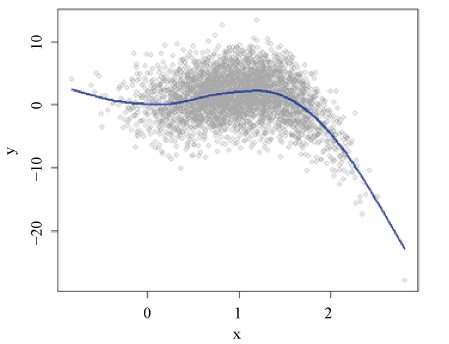Figure 1. Smoothing spline

#R语言加载ISLR包，调出数据集，进行数据可视化(如图2)

>library(ISLR)

>attach(Wage)

>plot(age,wage,xlim=agelims,cex=.5,col=darkgrey)Figure 2. Scatter diagram

5.1. 多项式回归模型

#运用交叉验证法选择多项次最佳回归次数

>nrow(Wage)

 3000

>train = sample(1:3000,2000)

>cv.err = vector(numeric,5)

>for(i in 1:5){

+ fit = lm(wage~poly(age,i),data = Wage,subset = train)

+ pred = predict(fit,newdata=Wage[-train,])

+ cv.err[i] = mean((pred-wage[-train])^2)

+}

>plot(1:5,cv.err,type=l)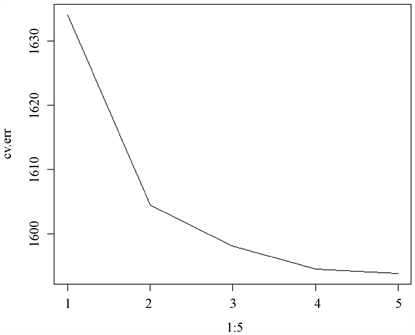Figure 3. Cross validation diagram

#构造4次多项式回归模型

>fit = lm(wage~poly(age,4),data = Wage)

>agelims = range(age)

>age.grid = seq(from=agelims,to=agelims)

>preds = predict(fit,newdata = list(age=age.grid),se=T)

>se.bands=cbind(preds$fit+2*preds$se.fit,preds$fit-2*preds$se.fit)# 构建预测值的置信区间

>plot(age,wage,xlim=agelims,cex=0.5,col=darkgrey)

>title(Degree-4 Polynomial,outer = T)

>lines(age.grid,preds$fit,lwd=2,col=blue) # 多项式回归预测曲线 >matlines(age.grid,se.bands,lwd=2,col = red,lty=3) # 置信区间曲线 图4中，红色虚线代表置信区间曲线，由拟合的图形可以看出，拟合的效果还不错。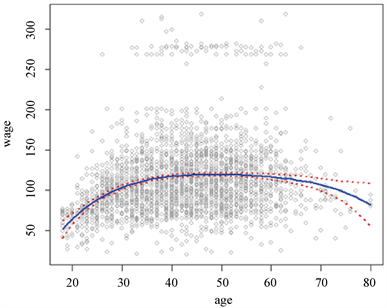Figure 4. Degree-4 polynomial regression 图4. 4次多项式回归 #方差分析 > anova(fit)Analysis of Variance Table Response: wage Df Sum Sq Mean Sq F value Pr(>F) poly(age, 4) 4 450482 112620 70.689 <2.2e−16 *** Residuals 2995 4771604 1593 --- Signif. codes:0 ‘***’ 0.001 ‘**’ 0.01 ‘*’ 0.05 ‘.’ 0.1 ‘ ’ 1 方差分析结果中，F值较大，回归模型显著，同样说明4次多项式回归拟合Wage-age的关系效果良好。 5.2. 光滑样条回归 光滑样条的拟合用R语言的smoothing.spline()实现。和多项式回归要确定次数一样，光滑样条回归的关键是要确定样条结点的个数、位置和光滑参数。 #光滑样条回归 >plot(age,wage,xlim=agelims,cex=.8,col=darkgrey) >title(Smoothing Spline) >fit=smooth.spline(age,wage,df=18)#第一次设定自由度为18 >fit2=smooth.spline(age,wage,cv=TRUE)#交叉验证法调整自由度 >fit2$df

 6.794596#调整后的自由度为6.7946

>lines(fit,col=red,lwd=2)

>lines(fit2,col=blue,lwd=2)

>legend(topright,legend=c(18 DF6.8 DF),col=c(redblue),lty=1,lwd=2,cex=.8)

> library(splines, lib.loc=F:/R-3.5.1/library)

> attr(bs(age,df=6.7946)knots)#样条结点位置

20%40%60% 100%

32 39 46 80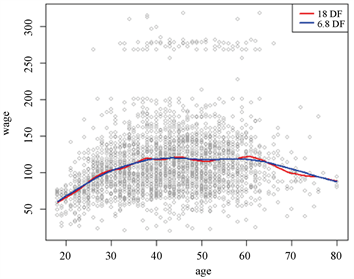Figure 5. Smoothing spline

5.3. 多项式回归与光滑样条比较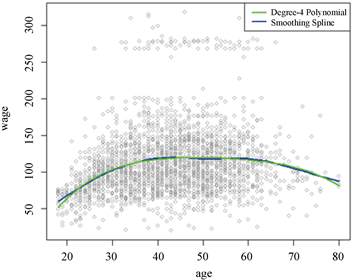Figure 6. Degree-4 polynomial VS smoothing spline

6. 结论

  陈生长, 徐勇勇, 夏结来. 光滑样条非参数回归方法及医学应用[J]. 中国卫生统计, 1999(6): 23-26.  陈长生, 伍稚萍, 吴冰. 儿童生长曲线的光滑样条非参数回归方法构建[J]. 北华大学学报(自然科学版), 2001, 2(3): 194-196.  卢一强, 陈中威. 部分线性模型的光滑样条推断[J]. 山西大同大学学报(自然科学版), 2010, 26(1): 1-4.  宋向东. 非参数回归的罚样条算法[J]. 燕山大学学报, 2007, 31(3): 263-265.